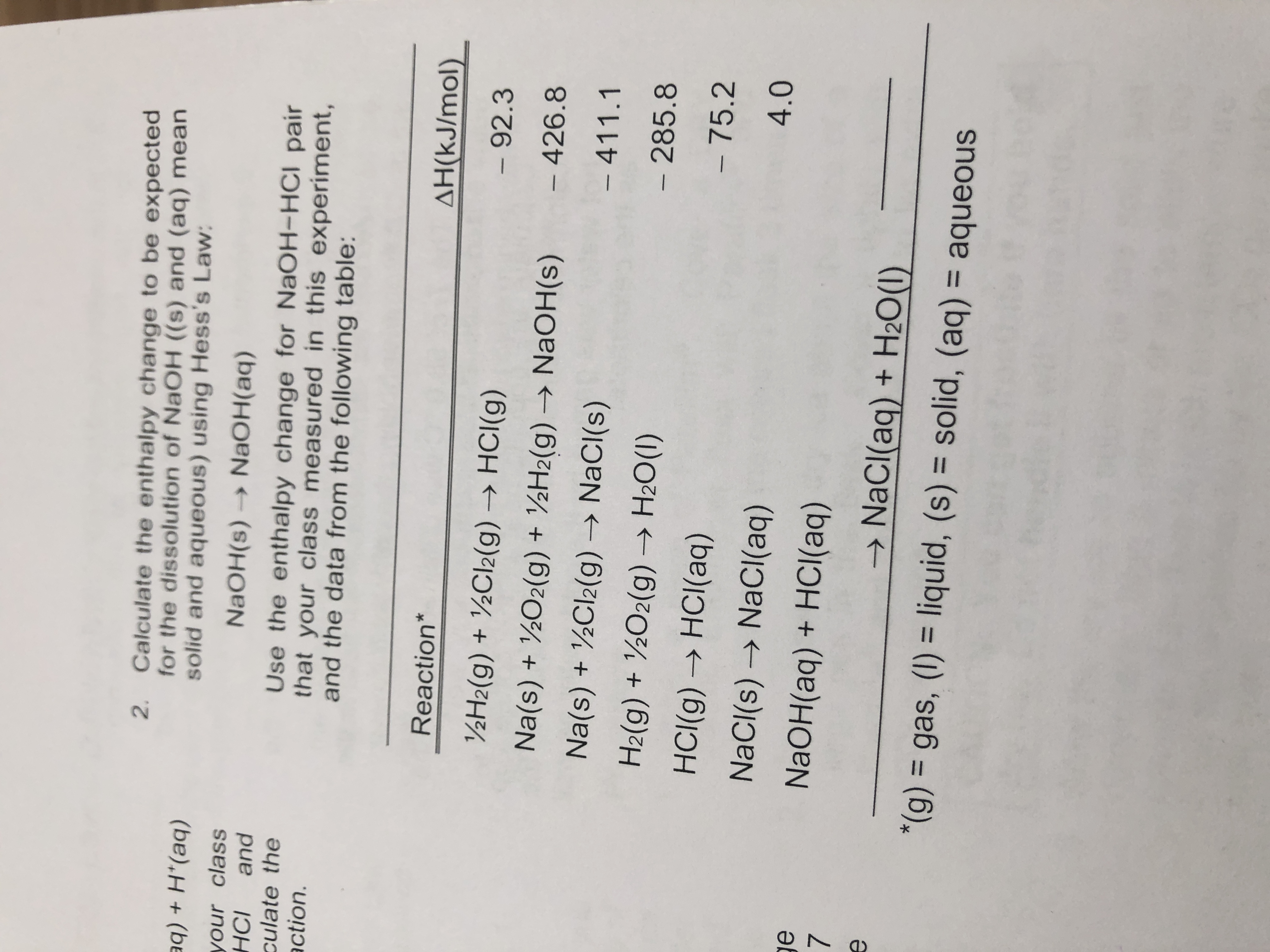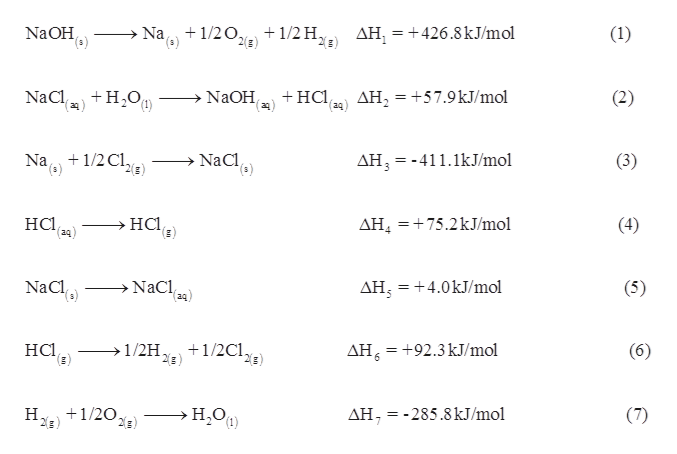# 2. Calculate the enthalpy change to be expectedfor the dissolution of NaOH ((s) and (aq) meansolid and aqueous) using Hess's Law:q + H*(aq)your classandNaOH(s) → NAOH(aq)НСІculate theaction.Use the enthalpy change for NaOH-HCI pairthat your class measured in this experiment,and the data from the following table:Reaction*AH(kJ/mol)½H2(g) + ½CI2(g) → HCI(g)- 92.3Na(s) + ½O2(g) + ½H2(g) → NaOH(s)–426.8Na(s) + ½CI2(g) → NaCI(s)– 411.1H2(g) + ½O2(g) → H2O(1)- 285.8HCI(g) → HCI(aq)-75.2NaCI(s) → NaCI(aq)4.0NaOH(aq) + HCI(aq)→ NaCl(aq) + H2O(1)*(g) = gas, (1) = liquid, (s) = solid, (aq) = aqueous%3D%3D%3D

Question
48 viewshelp_outlineImage Transcriptionclose2. Calculate the enthalpy change to be expected for the dissolution of NaOH ((s) and (aq) mean solid and aqueous) using Hess's Law: q + H*(aq) your class and NaOH(s) → NAOH(aq) НСІ culate the action. Use the enthalpy change for NaOH-HCI pair that your class measured in this experiment, and the data from the following table: Reaction* AH(kJ/mol) ½H2(g) + ½CI2(g) → HCI(g) - 92.3 Na(s) + ½O2(g) + ½H2(g) → NaOH(s) –426.8 Na(s) + ½CI2(g) → NaCI(s) – 411.1 H2(g) + ½O2(g) → H2O(1) - 285.8 HCI(g) → HCI(aq) -75.2 NaCI(s) → NaCI(aq) 4.0 NaOH(aq) + HCI(aq) → NaCl(aq) + H2O(1) *(g) = gas, (1) = liquid, (s) = solid, (aq) = aqueous %3D %3D %3D fullscreen
check_circle

star
star
star
star
star
1 Rating
Step 1

According to Hess’s law, the overall enthalpy change of the reaction is the sum...help_outlineImage TranscriptioncloseNa, +1/20 = +426.8kJ/mol NaOH,) + 1/2 Hy ΔΗ - (1) (), (s) +HCl 39) (24) NaCl +H,O, NaOH, (2) AH, = +57.9kJ/mol (1) Na, +1/2 Cl,, NaCl,) AH, = -411.1kJ/mol (3) 2{z) на -нO НCі, AH, =+75.2kJ/mol (4) -4 (24) NaCl,) NaCl, (24) AH, =+4.0kJ/mol (5) 1/2HE) ΔΗ нCl (6) +1/2Cl.. +92.3 kJ/mol н.О) +1/20, →H,O, ΔΗ = - 285.8 kJ/mol (7) (1) fullscreen

### Want to see the full answer?

See Solution

#### Want to see this answer and more?

Solutions are written by subject experts who are available 24/7. Questions are typically answered within 1 hour.*

See Solution
*Response times may vary by subject and question.
Tagged in

### Chemical Thermodynamics